Calculator Installation & Instructions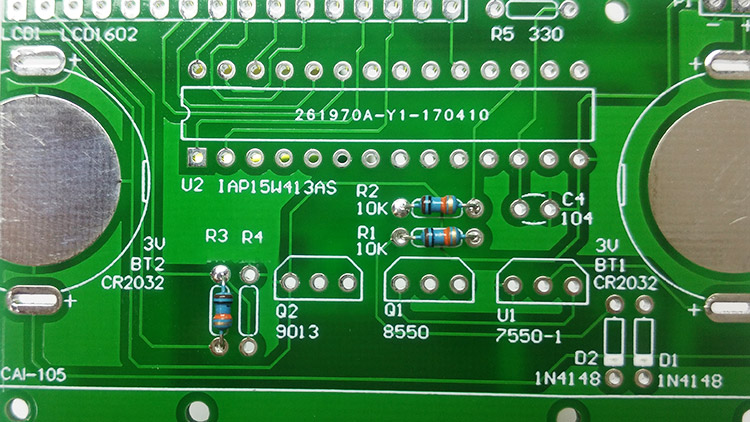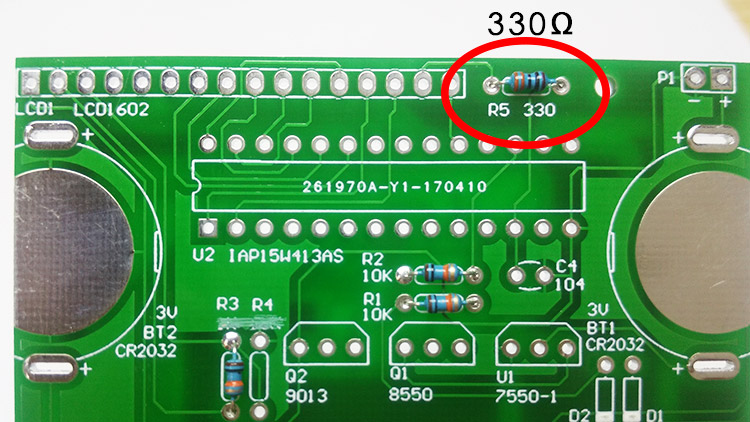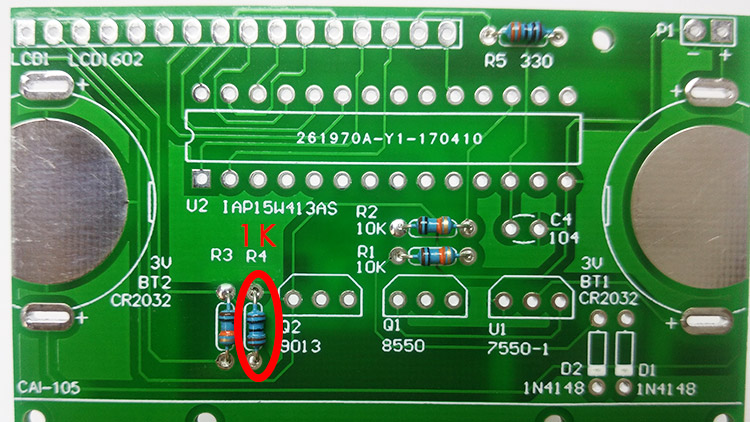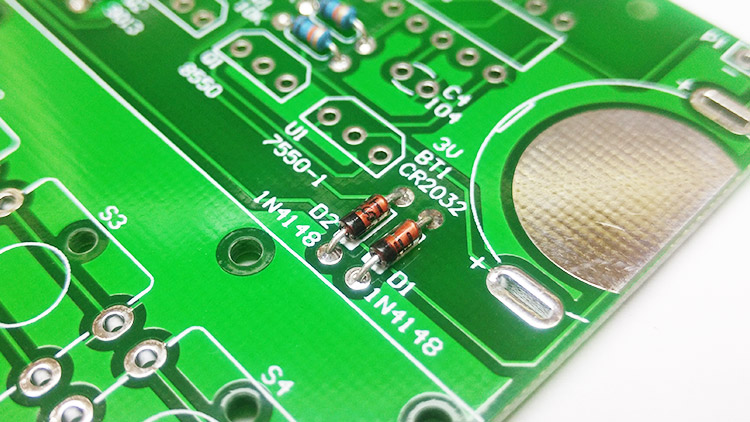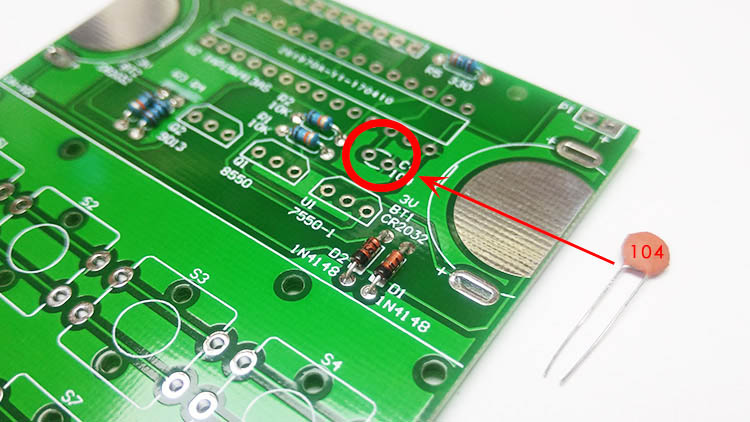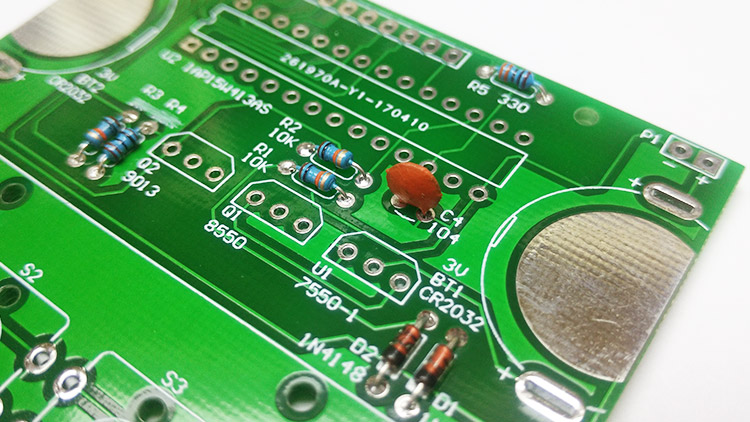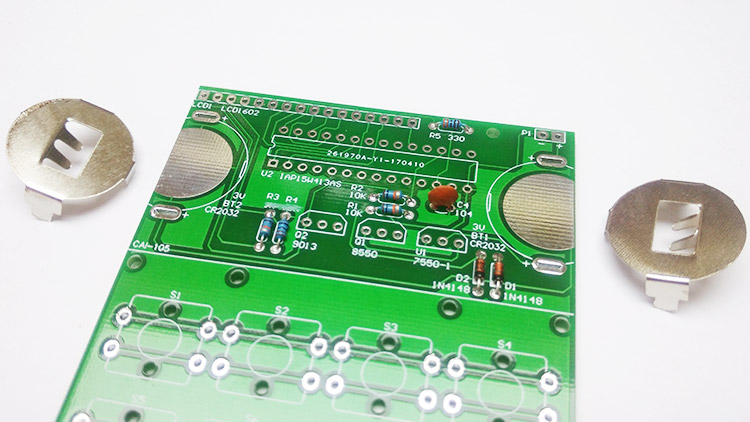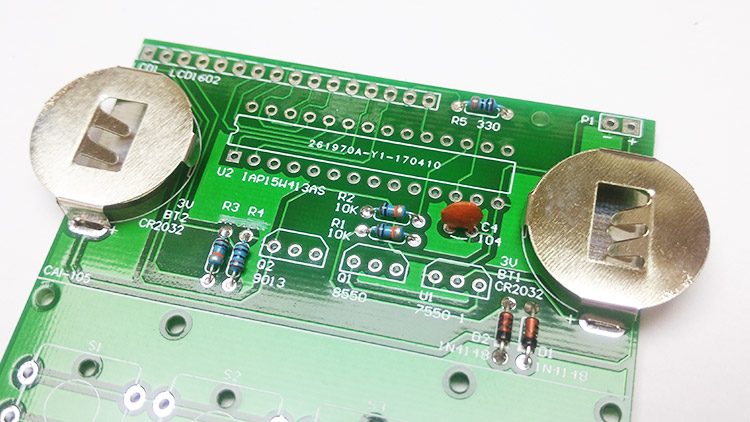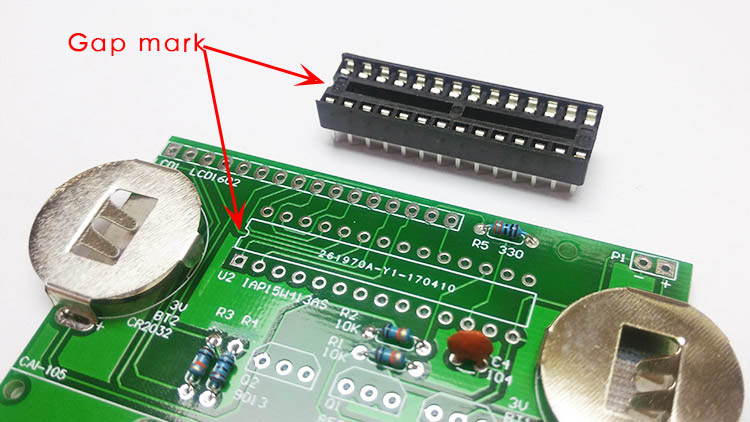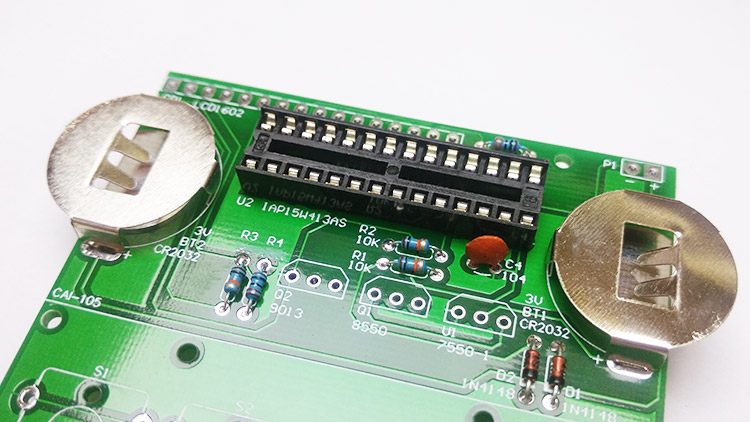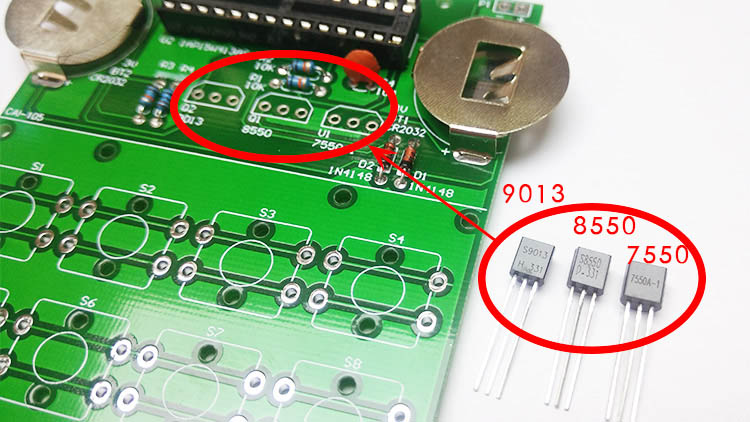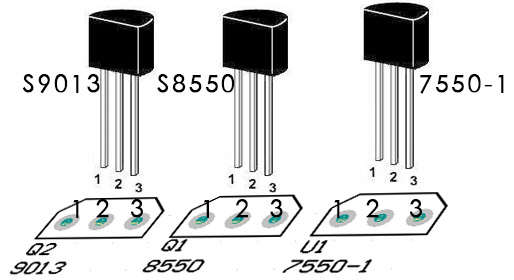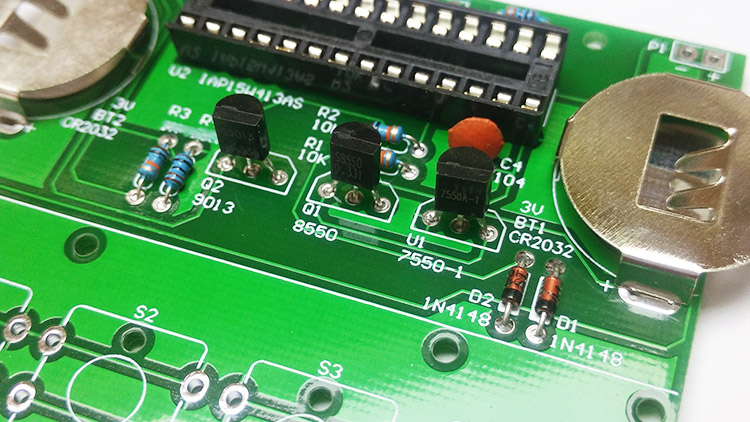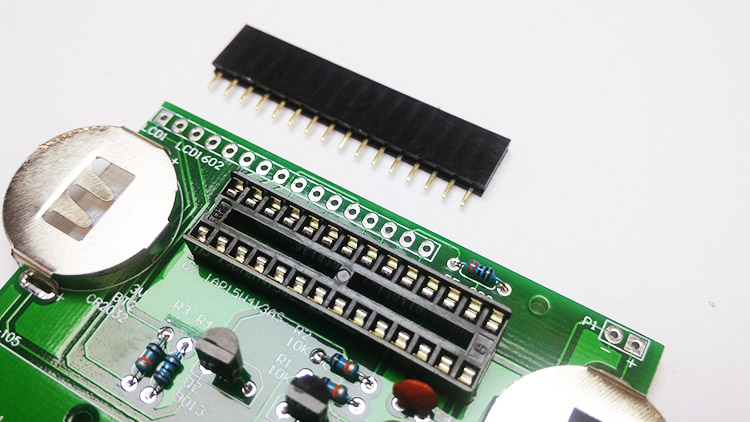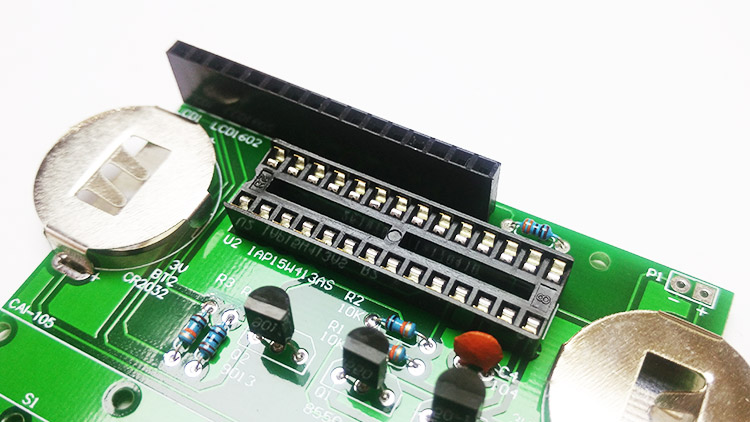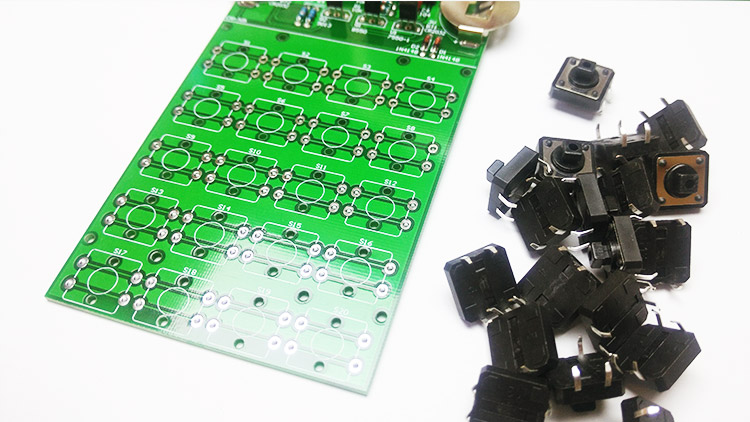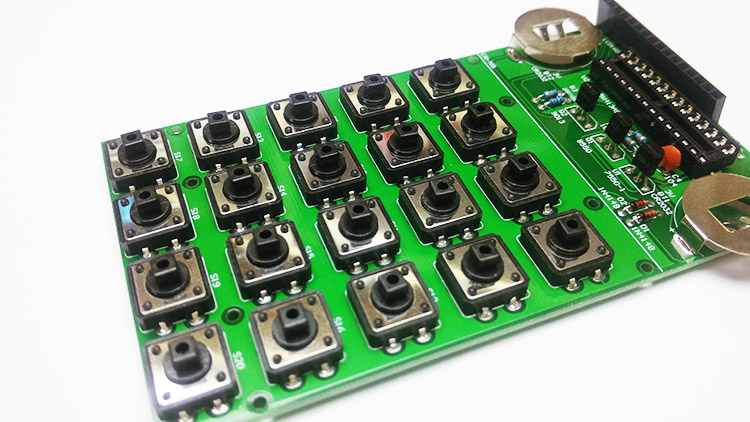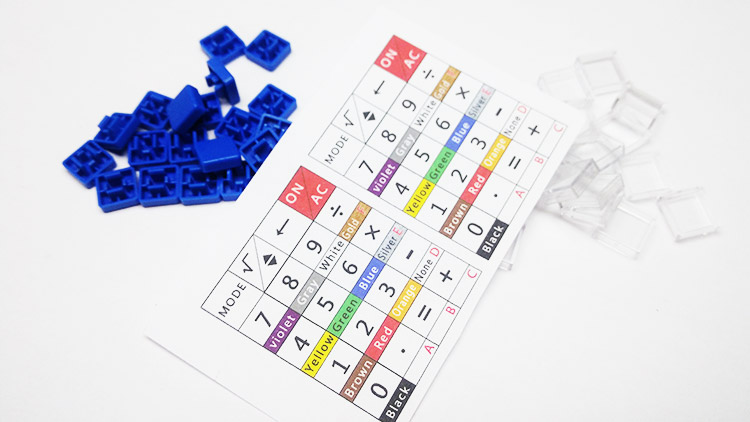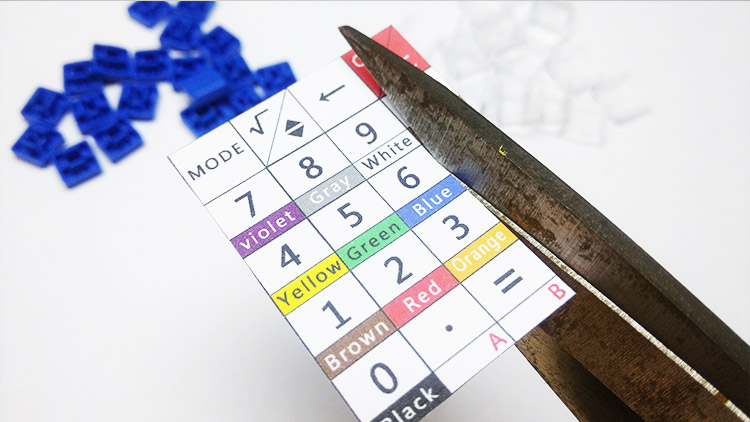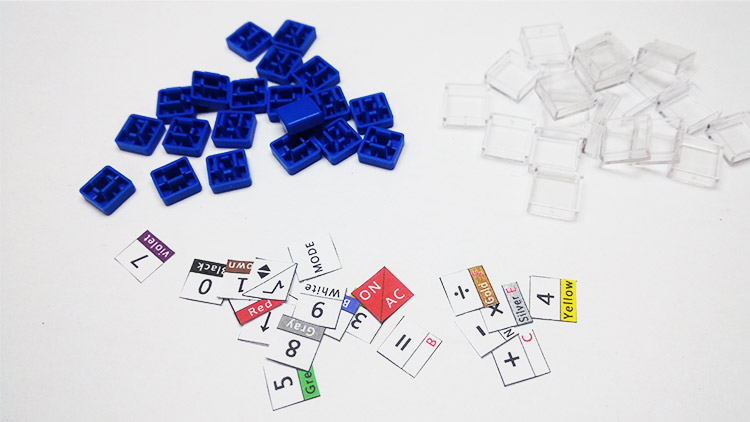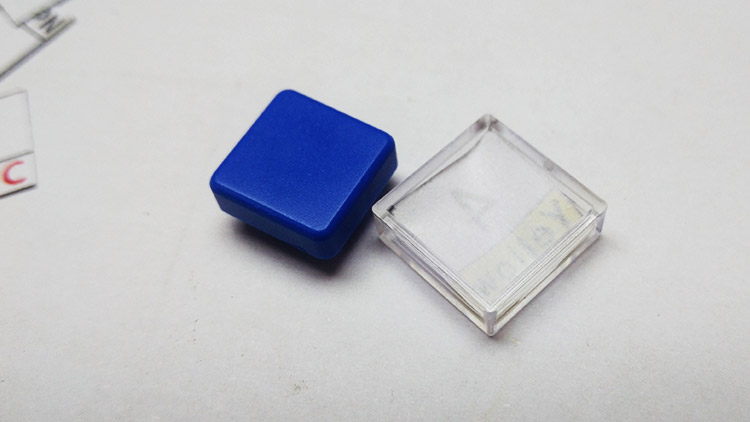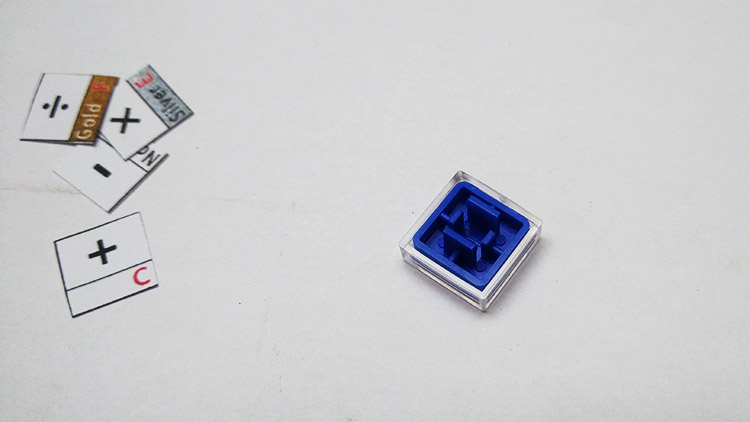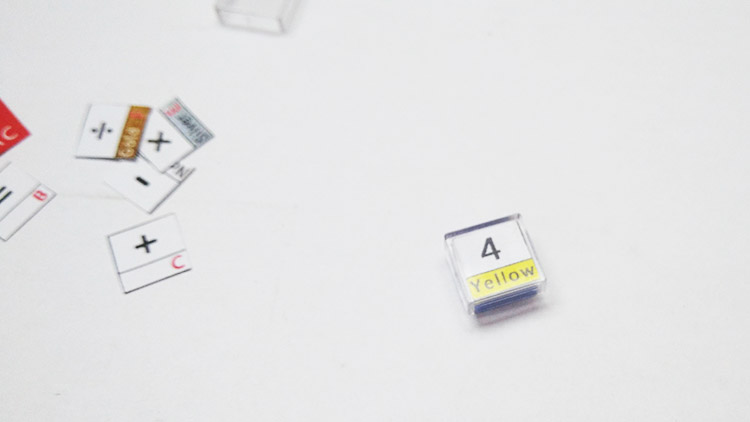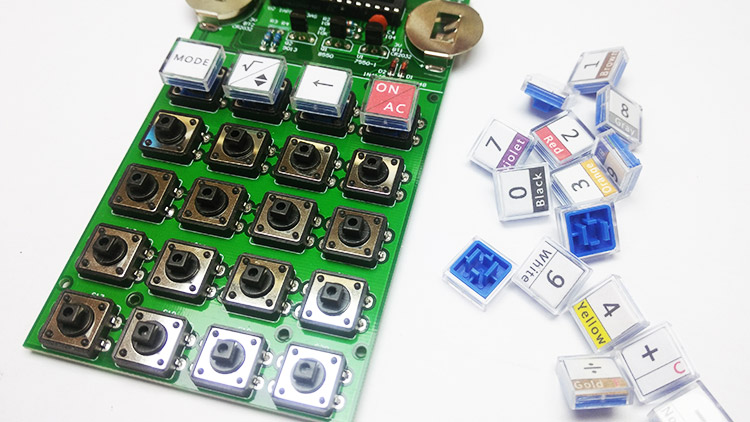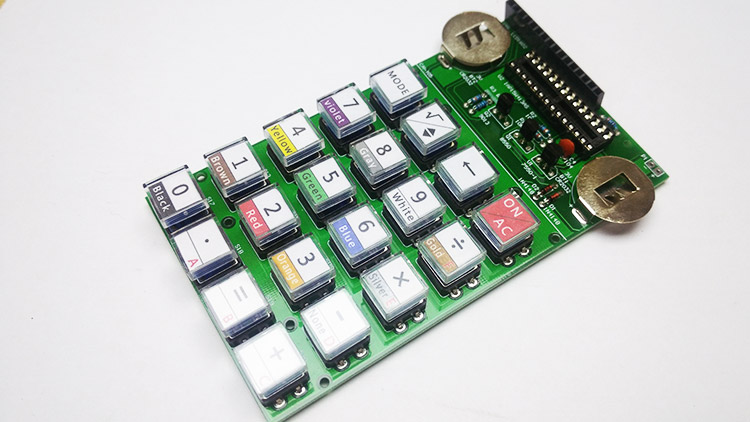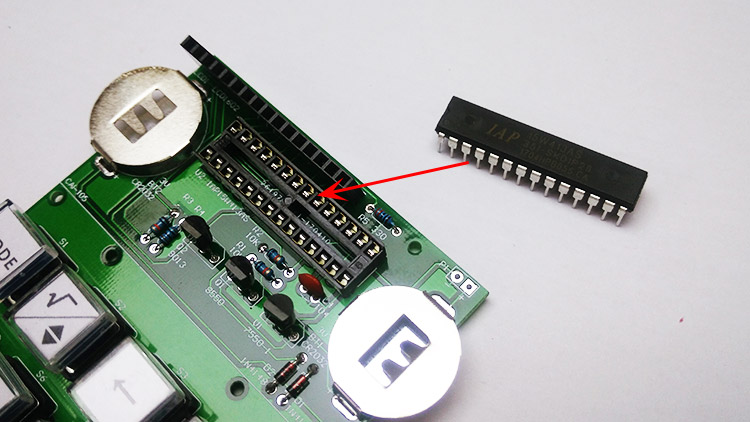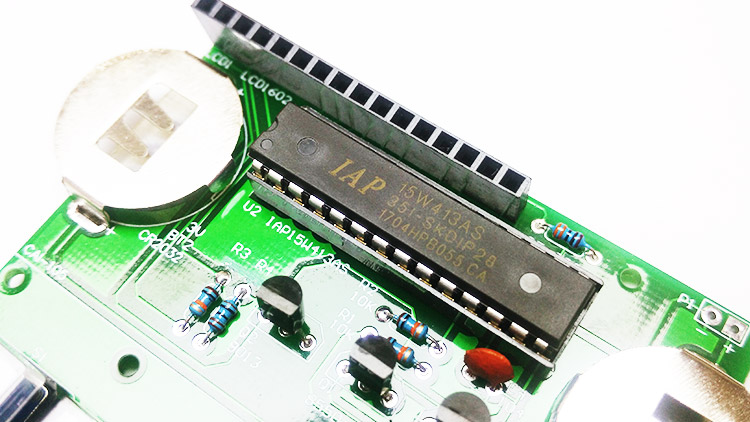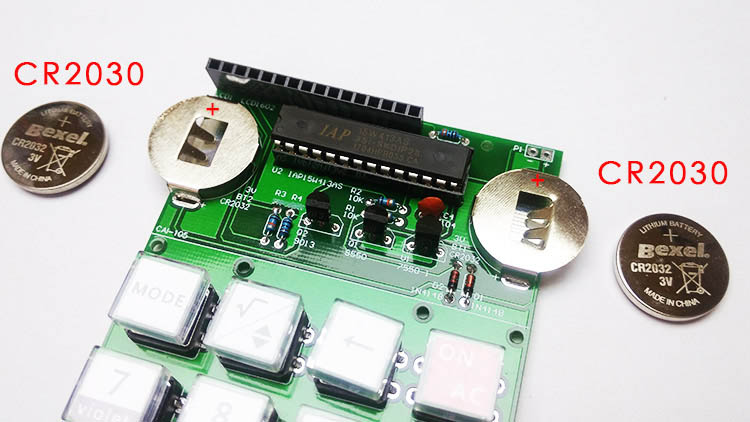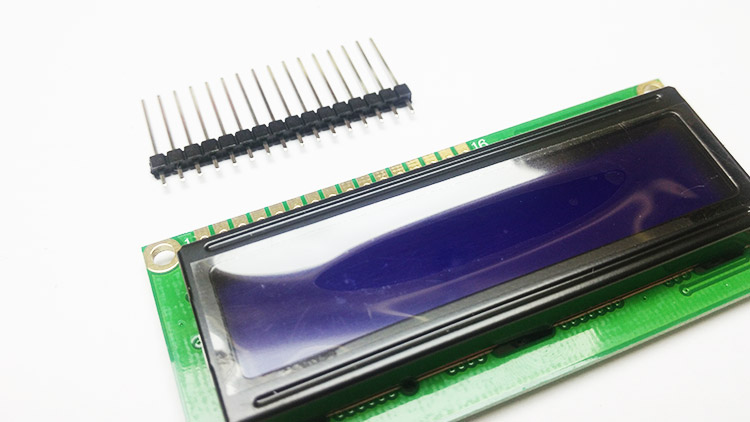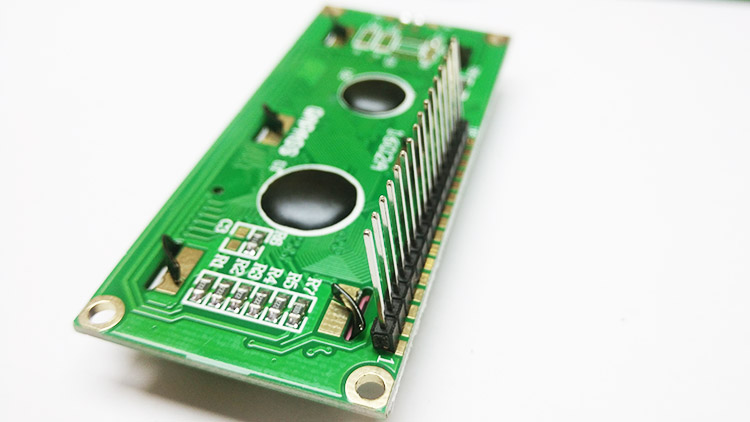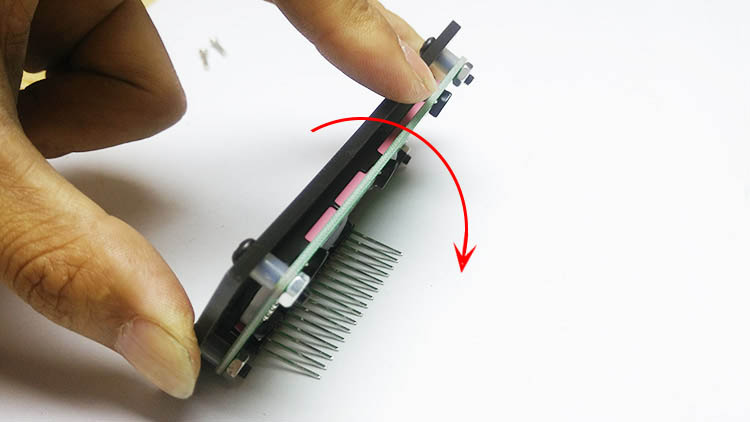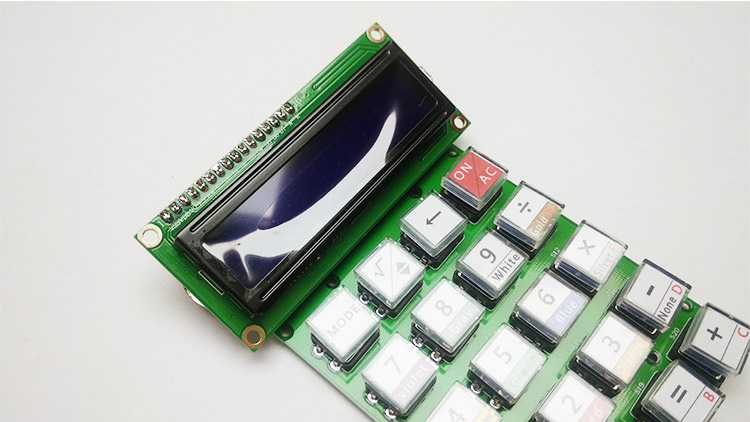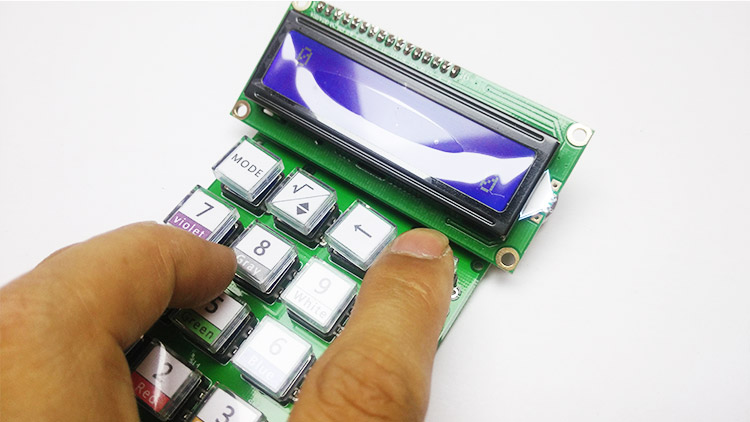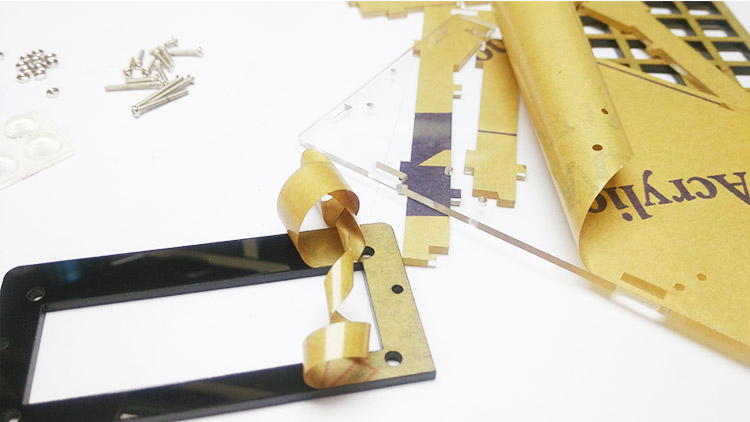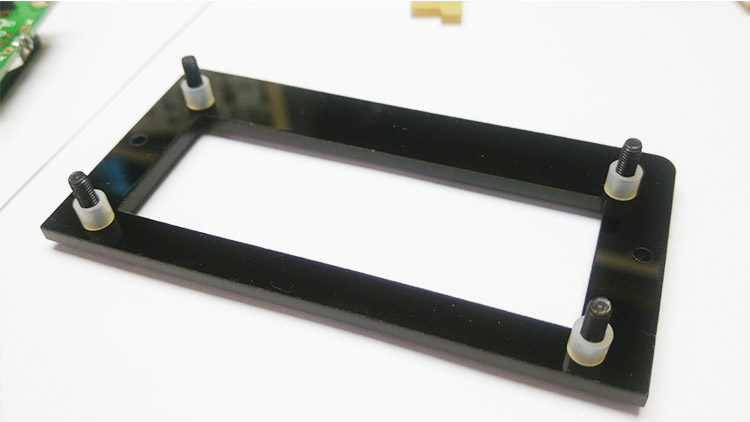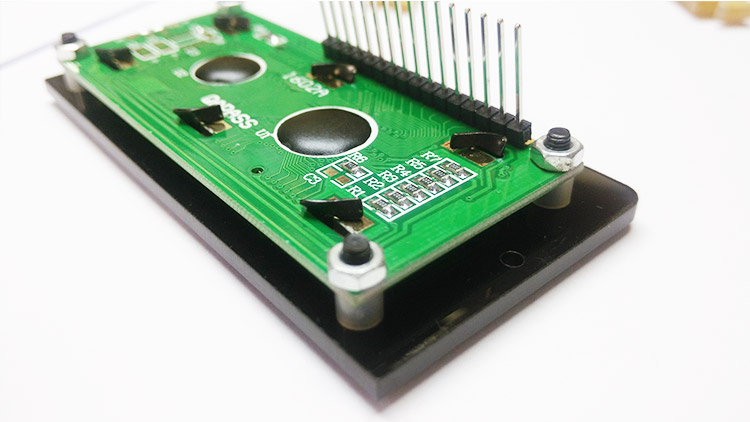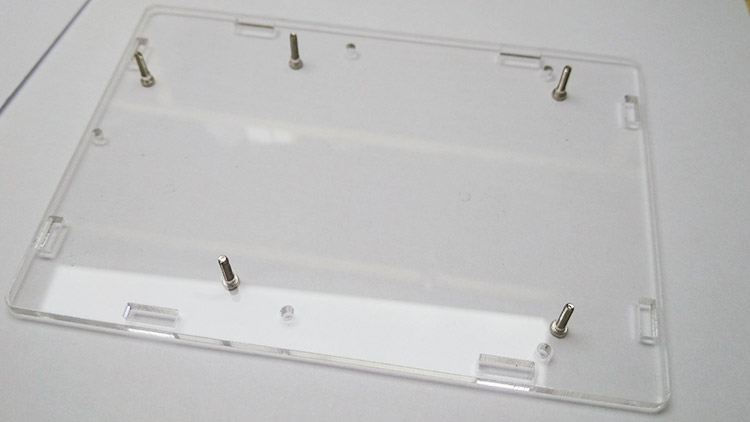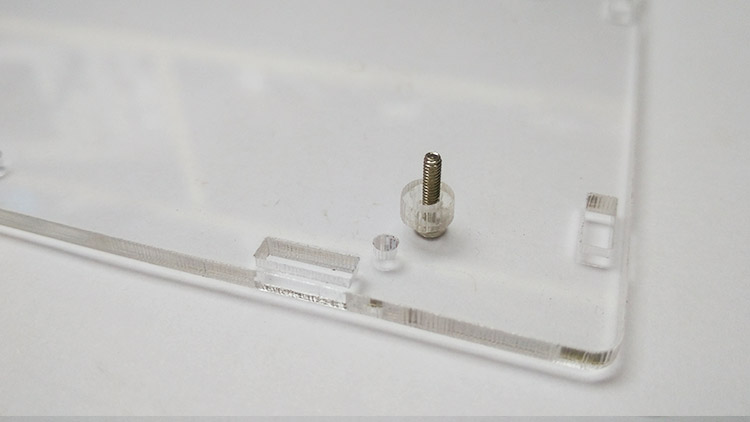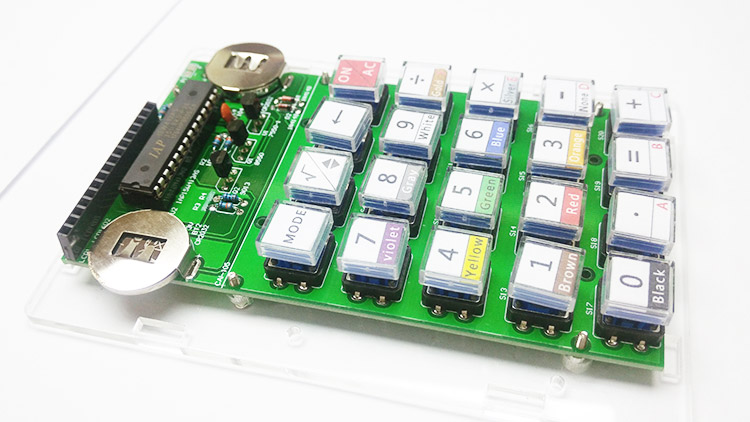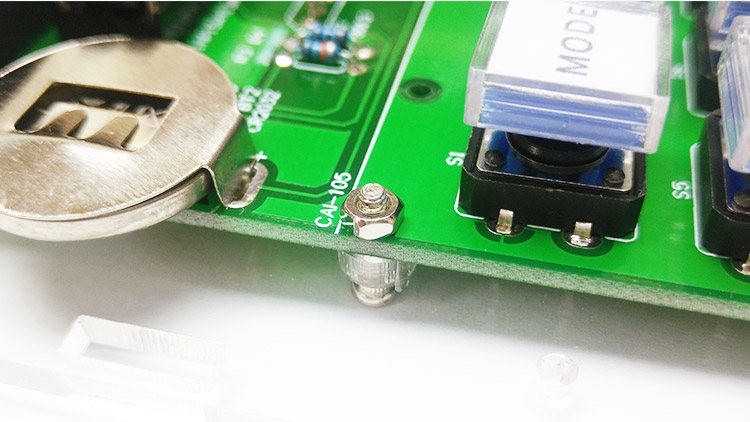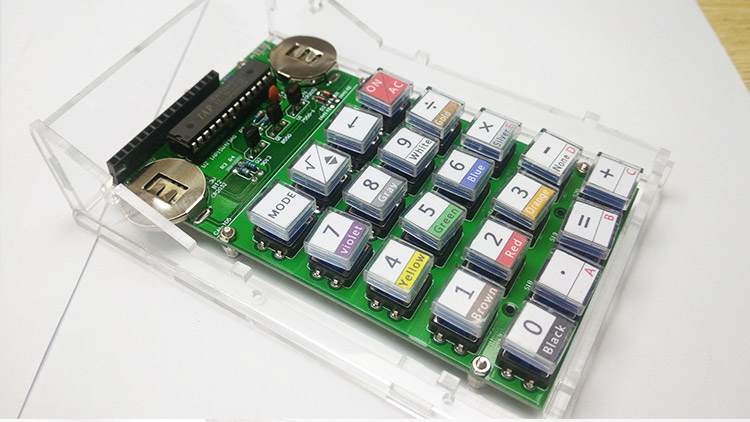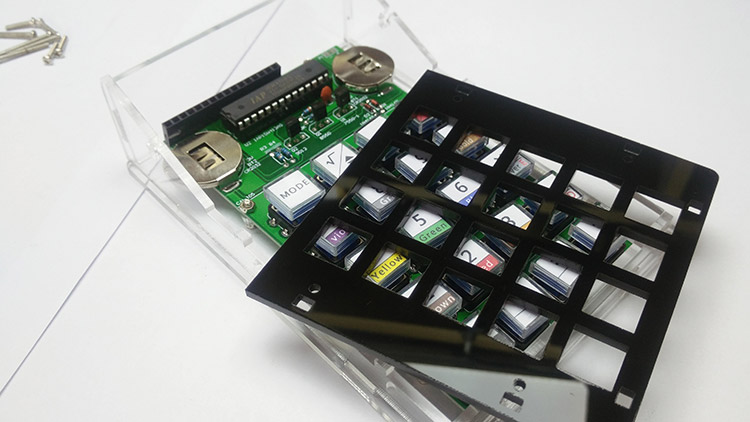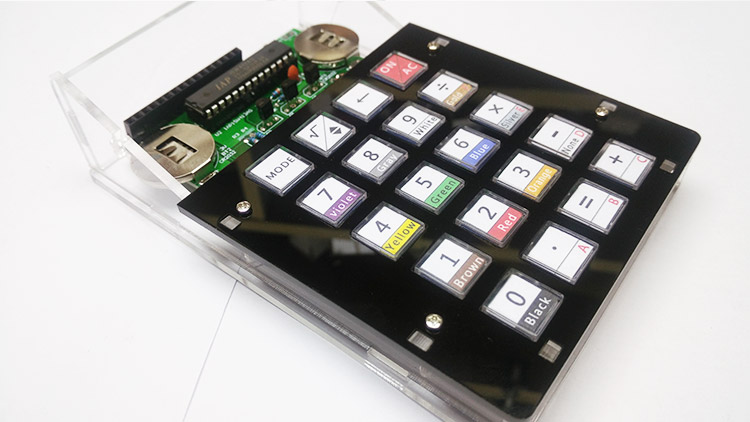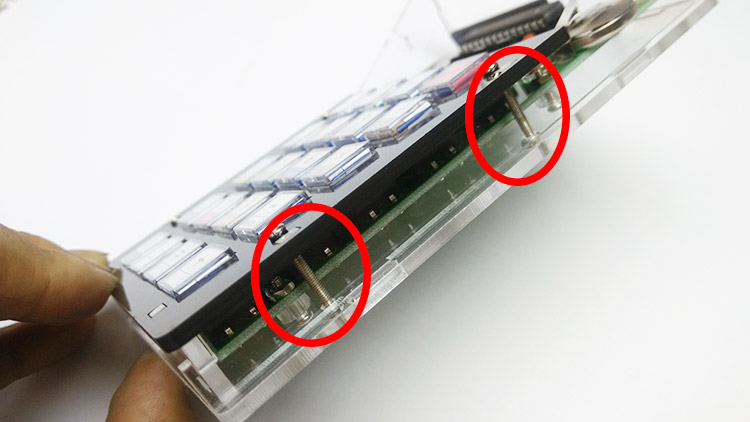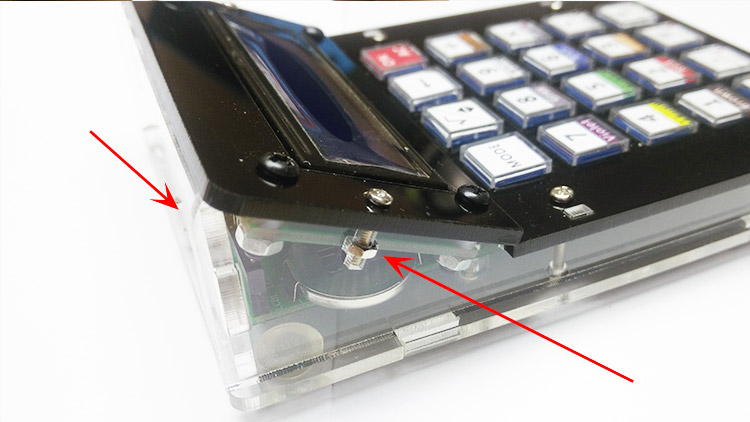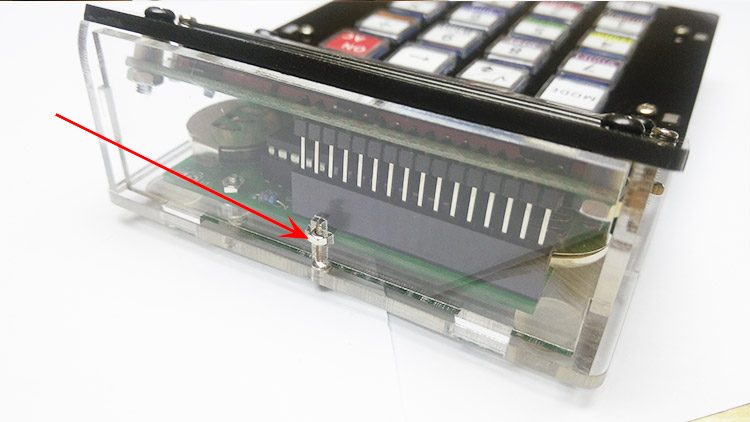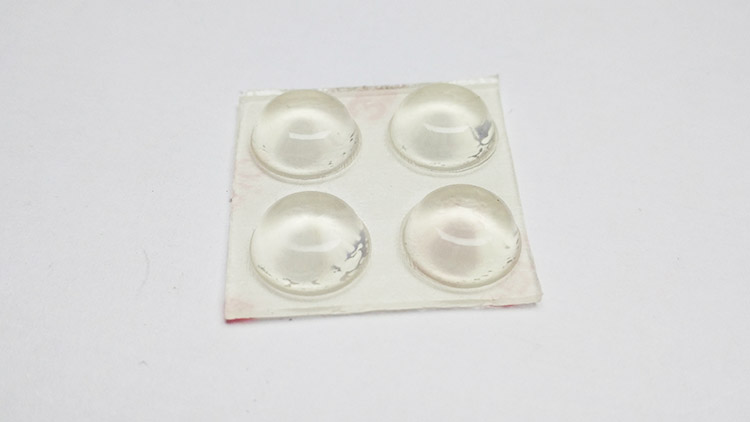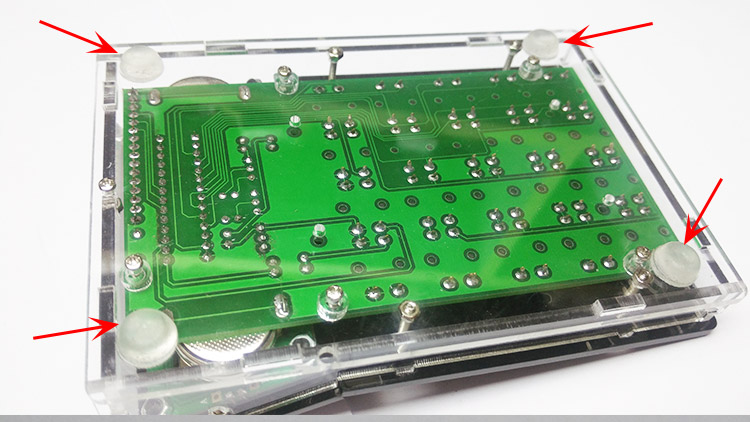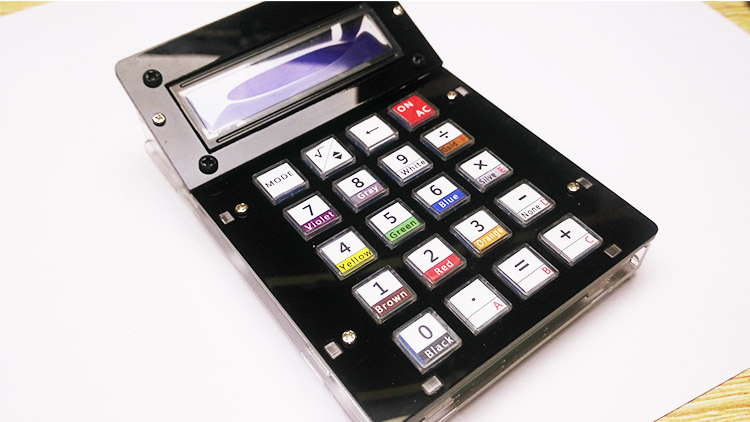Calculator Installation & Instructions Installation of Components Weld corresponding components onto the circuit board according to the reference picture. Install short components such as resistor and IN4148 firstly and then high components. Install IC base and then place IC on the IC base. Power supply: 2 CR1220 batteries; press ON to power it on; the calculator automatically powers off after 30 seconds of idleness. Circuit schematic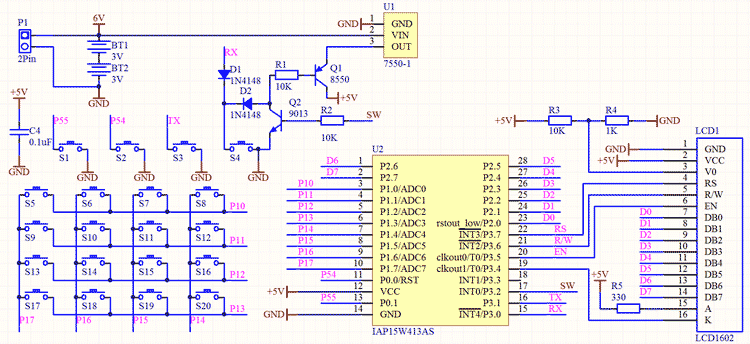Component layout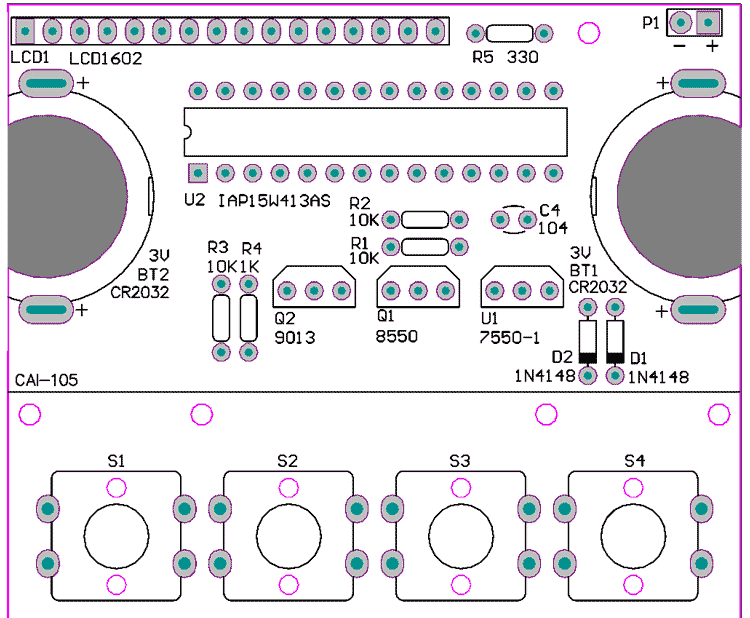Function 1: Decimal arithmetic operation, with decimal points and negative number calculation E.g.: The calculation of 5*6=30 How to operate: Press ON to power it on; press 5, *, 6, and then ?= ?in sequence, and then you have the result = 25. Press ON/AC to reset. E.g.: The calculation of -8+2=-6 How to operate: Press ON/AC to power it on; press ?- , ?8 , ?+ , ?2 , and then ?= ?in sequence, and then you have the result = -6. Press ON/AC to reset. Function 2: Calculation of four-ring resistance or 5-ring resistance. Enter the colors of color rings in turn to obtain resistance value and error E.g.: 5-ring resistance: yellow; violet; black; brown; brown. The resistance is 4700Ω. How to operate: Press ON/AC to power it on; press MODE to switch to the Color Ring R C 5 for the calculation of 5-ring resistance. Press Yellow, press Violet, press Black, press Brown, and press Brown. Then you have the resistance value R=4700, and the error is 1%. E.g.: 4-ring resistance: Green; red; yellow; gold. The resistance is 520Ω. How to operate: Press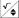?one more time while you are in the Color Ring R C 5 to switch to Color Ring R C 4 for the calculation of 4-ring resistance. Press Green, press Red, press Yellow, and press Gold. Then you have the resistance value R=520, and the error is 5%. Press ON/AC to reset. Press MODE to select other functions. Function 3: If the power supply voltage, working voltage and working current of LED are known, then how to evaluate the value of LED’s series resistance Formula: Vi-Vd means the power supply voltage minus the working voltage of LED. I is the working current of LED E.g.: Suppose that the power supply voltage (Vi) is 5V, the working voltage of LED (Vd) is 3V, and Vi-Vd=2V, and the working current of LED (I) is 10mA, what is the value of the series resistance R? How to operate: Press ON/AC to power it on; press MODE to switch to the interface Rled? Vi-Vd=?? V? I=?? mA??? R=? Press ?2 ?(Vi-Vd=2V); press?to switch to enter the current; press ?10 ?(the working current of LED); press ?= ?. The value of the series resistance is obtained and it is 200Ohm. Press ON/AC to reset. Press MODE to select other functions. Function 4: The conversion from decimal number - hexadecimal number; the conversion from hexadecimal number - decimal number How to operate: Press ON/AC to power it on, and press MODE to switch to the 10HEX-16HEX to convert decimal numbers to hexadecimal numbers. Enter a decimal number and a hexadecimal number is automatically obtained. Press ON/AC to reset. Press?to switch to the while you are in the 10HEX-16HEX to switch to the 16HEX-10HEX to convert hexadecimal numbers to decimal numbers. Enter a hexadecimal number and a decimal number is automatically obtained. Press ON/AC to reset. Press MODE to select other functions. Function 5: Square root E.g.: √4=2 How to operate: Press ON/AC to power it on, press?and √0 will occur; press ?4 ?and ?= ?in sequence, and you will obtain the result =2. Description of Keys: Mode: Mode selection: Square root key and switch key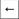: Backspace key which is used to eliminate mistakenly entered digits one by one. Clear key, which is used to clear all displayed results or entered content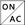?key: ON/OFF and reset key All number keys are used in a combined way and each key is divided into two sections. The parts above are the functions of normal calculator. The parts below marked with colors and texts are used for the calculation of color ring resistance. ABCDEF keys: Press 0-9 and A-F keys while converting number systems